# DAV Class 3 Maths Chapter 3 Worksheet 3 Solutions

The DAV Class 3 Maths Book Solutions and DAV Class 3 Maths Chapter 3 Worksheet 3 Solutions of Subtraction offer comprehensive answers to textbook questions.

## DAV Class 3 Maths Ch 3 WS 3 Solutions

Subtract:

Question 1.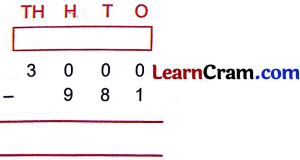Solution:Question 2.Solution:Question 3.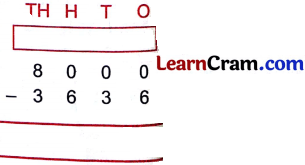Solution:### DAV Class 3 Maths Chapter 3 Worksheet 3 Notes

If the number have 0 at three placesStep – 1:
Borrow 1 thousand and change into 10 hundreds.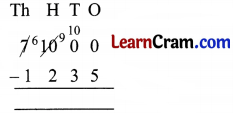Step – 2:
Borrow 1 hundred and change into 10 tens.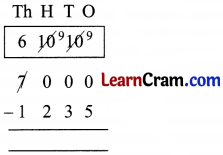Step – 3:
Borrow 1 ten and change into 10 ones.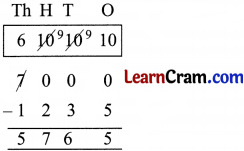Step – 4:
Now subtract